Скачать презентацию Are You Smarter Than a 3 rd Grader

d87033d115cfe04d778ca37d663e33e5.ppt

• Количество слайдов: 25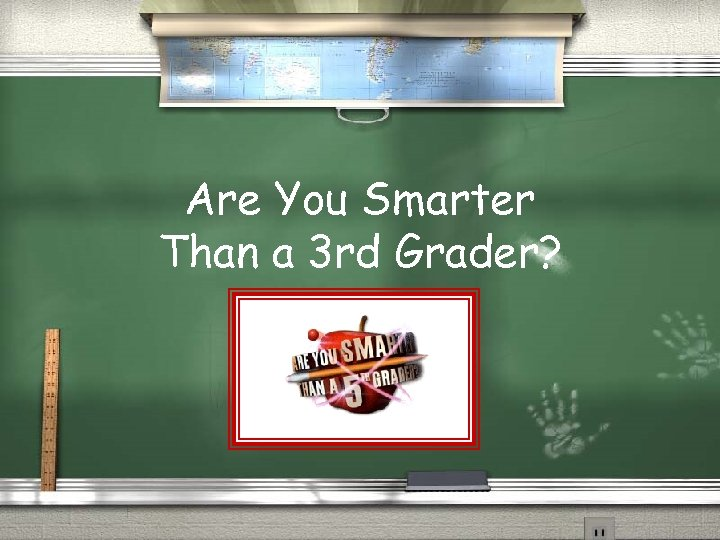Are You Smarter Than a 3 rd Grader?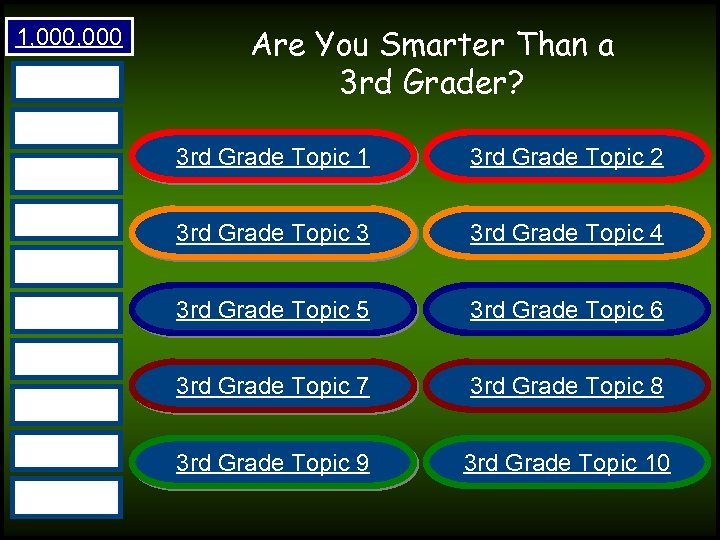1, 000 500, 000 Are You Smarter Than a 3 rd Grader? 300, 000 175, 000 100, 000 3 rd Grade Topic 1 3 rd Grade Topic 2 3 rd Grade Topic 3 3 rd Grade Topic 4 3 rd Grade Topic 5 3 rd Grade Topic 6 3 rd Grade Topic 7 3 rd Grade Topic 8 3 rd Grade Topic 9 3 rd Grade Topic 10 50, 000 25, 000 10, 000 5, 000 2, 000 1, 000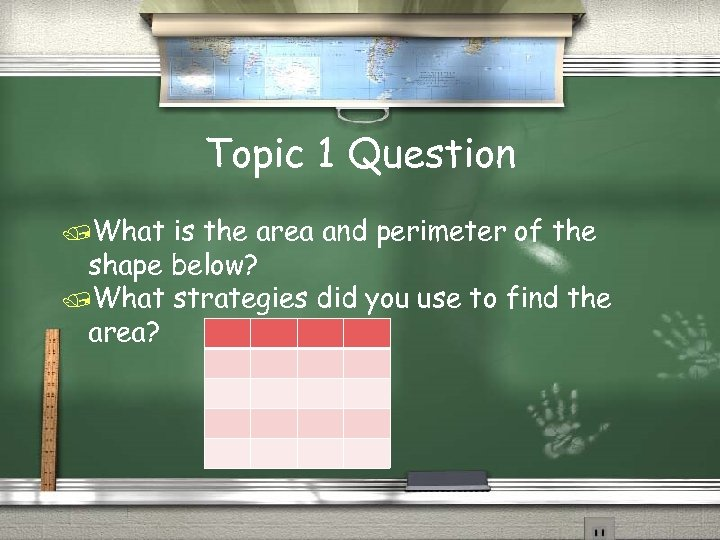Topic 1 Question /What is the area and perimeter of the shape below? /What strategies did you use to find the area?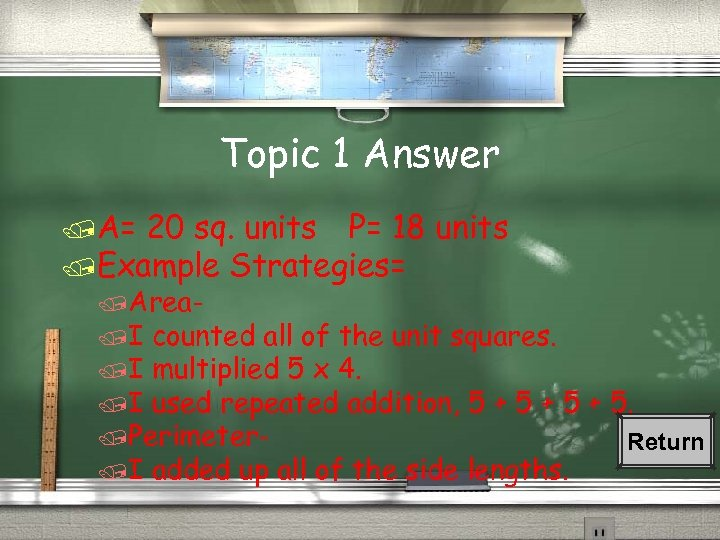Topic 1 Answer /A= 20 sq. units P= 18 units /Example Strategies= /Area/I counted all of the unit squares. /I multiplied 5 x 4. /I used repeated addition, 5 + 5 + 5. /Perimeter. Return /I added up all of the side lengths.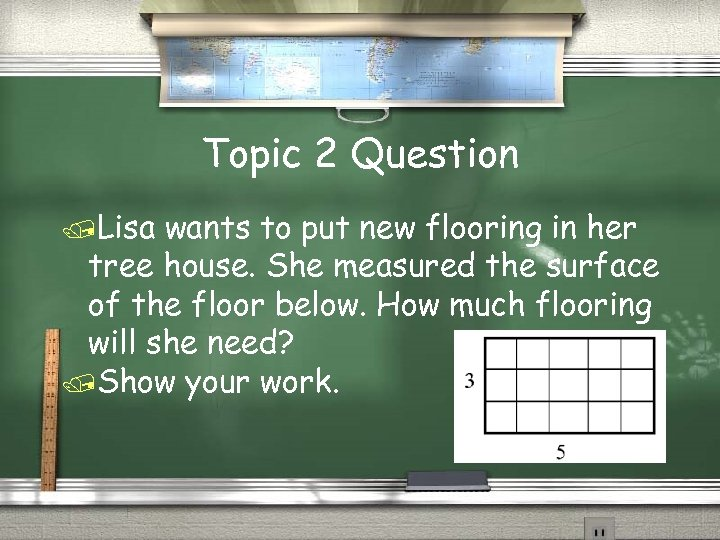Topic 2 Question /Lisa wants to put new flooring in her tree house. She measured the surface of the floor below. How much flooring will she need? /Show your work.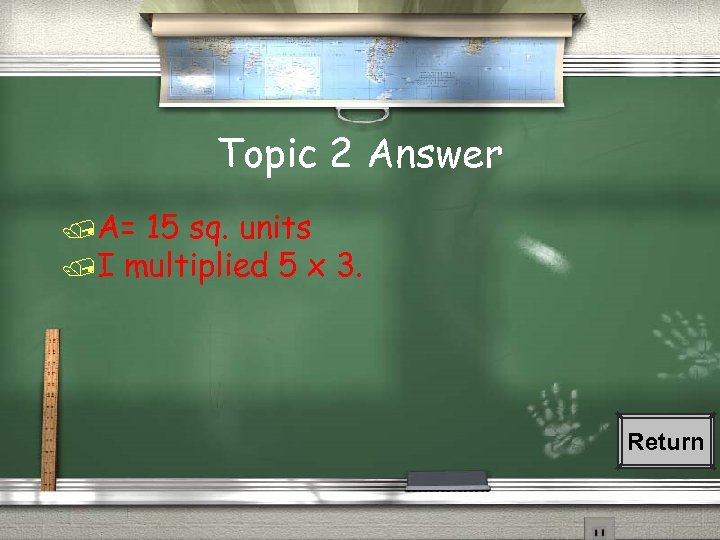Topic 2 Answer /A= 15 sq. units /I multiplied 5 x 3. Return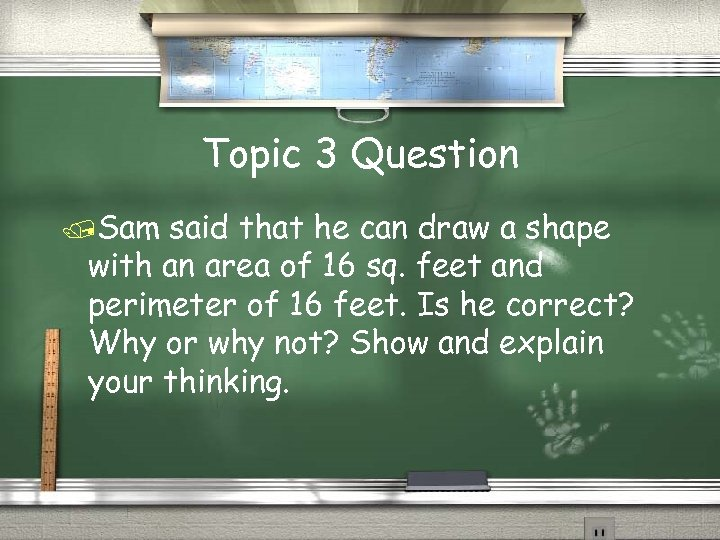Topic 3 Question /Sam said that he can draw a shape with an area of 16 sq. feet and perimeter of 16 feet. Is he correct? Why or why not? Show and explain your thinking.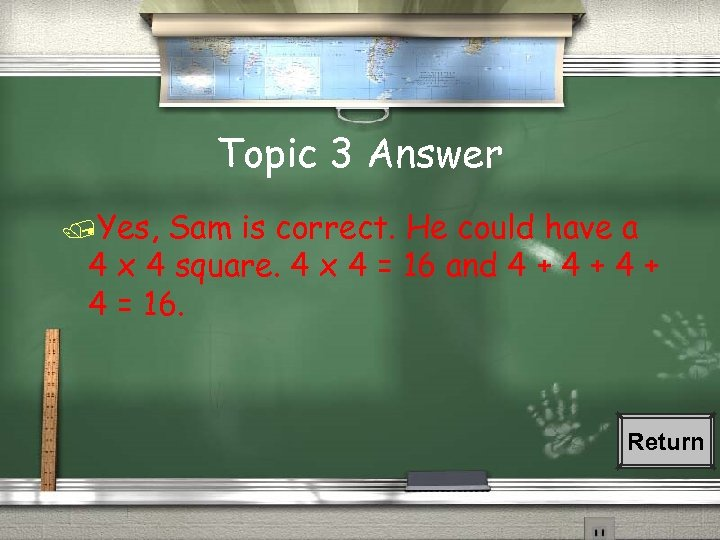Topic 3 Answer /Yes, Sam is correct. He could have a 4 x 4 square. 4 x 4 = 16 and 4 + 4 + 4 = 16. Return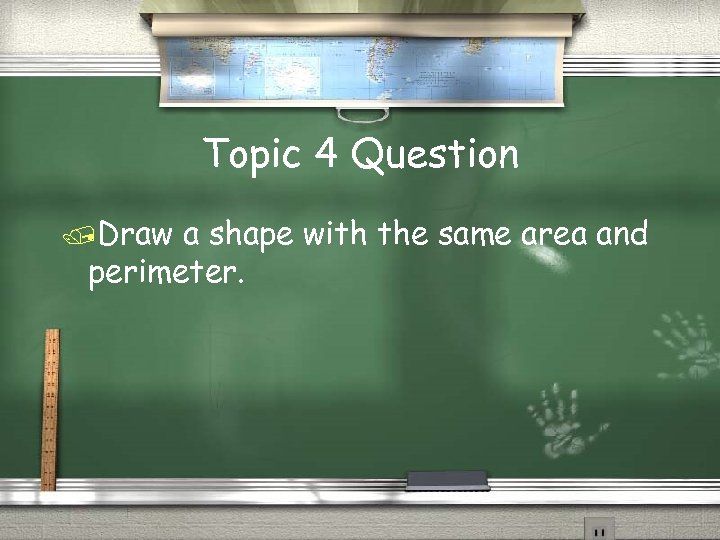Topic 4 Question /Draw a shape with the same area and perimeter.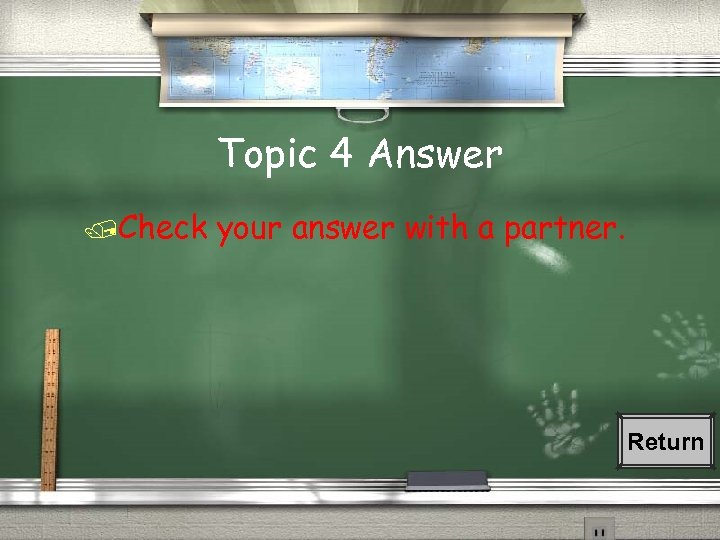Topic 4 Answer /Check your answer with a partner. Return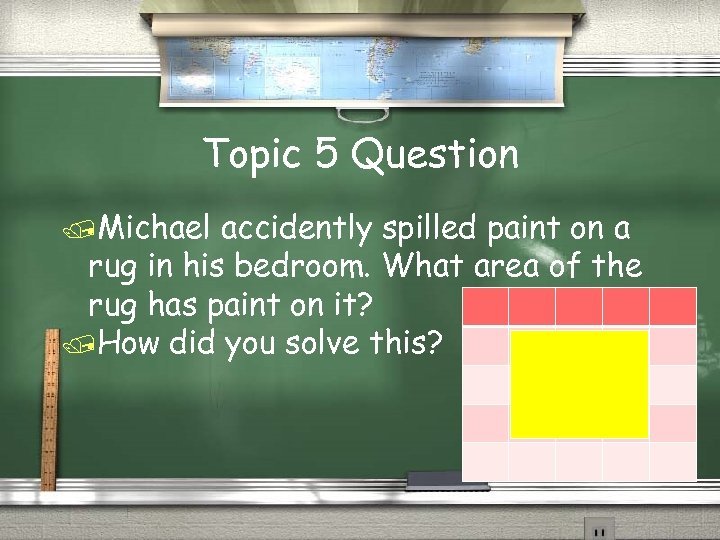Topic 5 Question /Michael accidently spilled paint on a rug in his bedroom. What area of the rug has paint on it? /How did you solve this?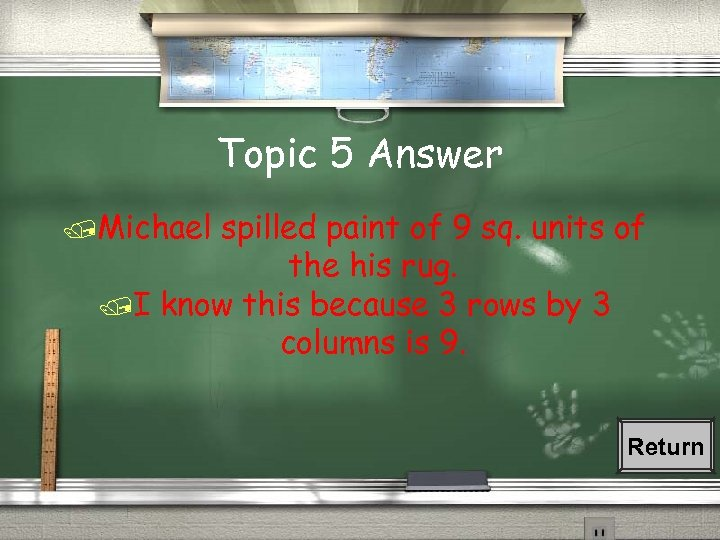Topic 5 Answer /Michael spilled paint of 9 sq. units of the his rug. /I know this because 3 rows by 3 columns is 9. Return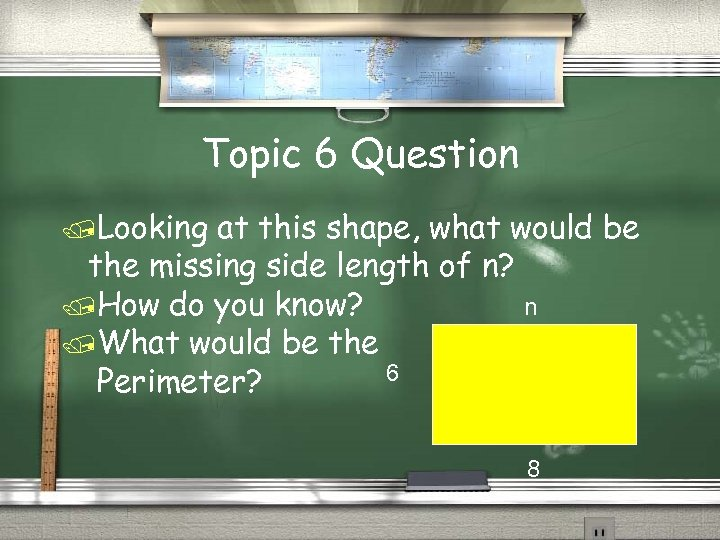Topic 6 Question /Looking at this shape, what would be the missing side length of n? n /How do you know? /What would be the 6 Perimeter? 8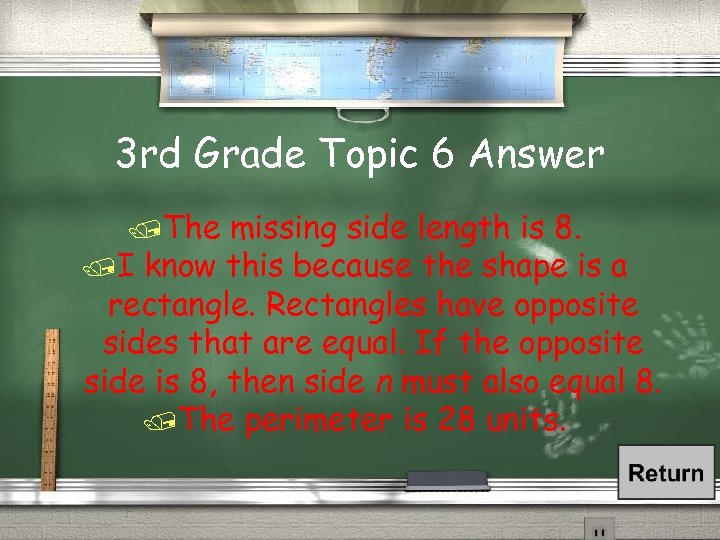3 rd Grade Topic 6 Answer /The missing side length is 8. /I know this because the shape is a rectangle. Rectangles have opposite sides that are equal. If the opposite side is 8, then side n must also equal 8. /The perimeter is 28 units.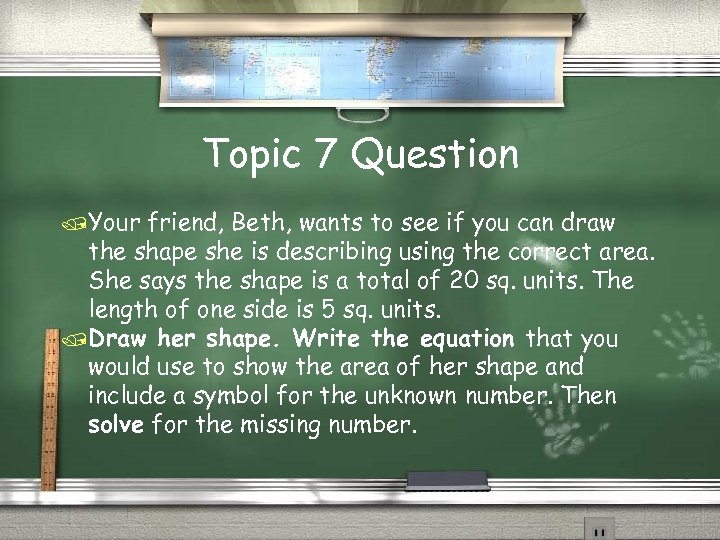Topic 7 Question /Your friend, Beth, wants to see if you can draw the shape she is describing using the correct area. She says the shape is a total of 20 sq. units. The length of one side is 5 sq. units. /Draw her shape. Write the equation that you would use to show the area of her shape and include a symbol for the unknown number. Then solve for the missing number.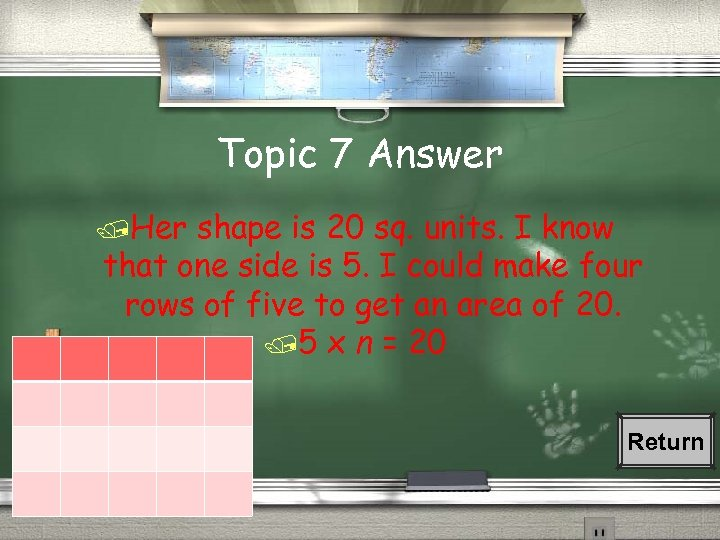Topic 7 Answer /Her shape is 20 sq. units. I know that one side is 5. I could make four rows of five to get an area of 20. /5 x n = 20 Return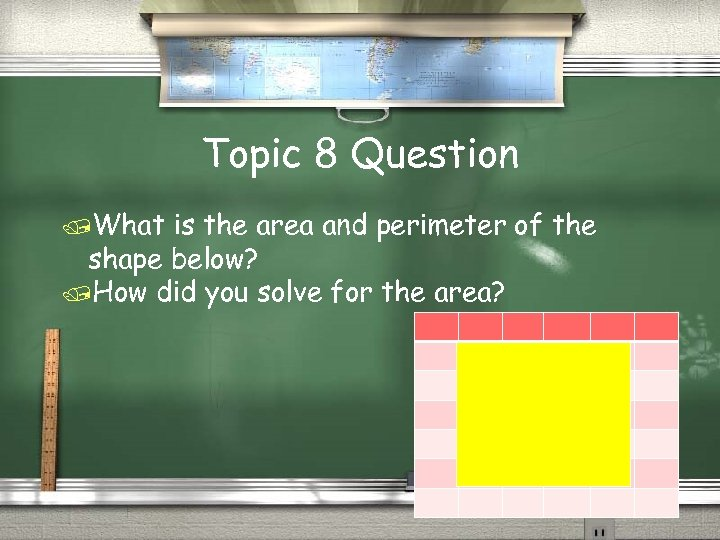Topic 8 Question /What is the area and perimeter of the shape below? /How did you solve for the area?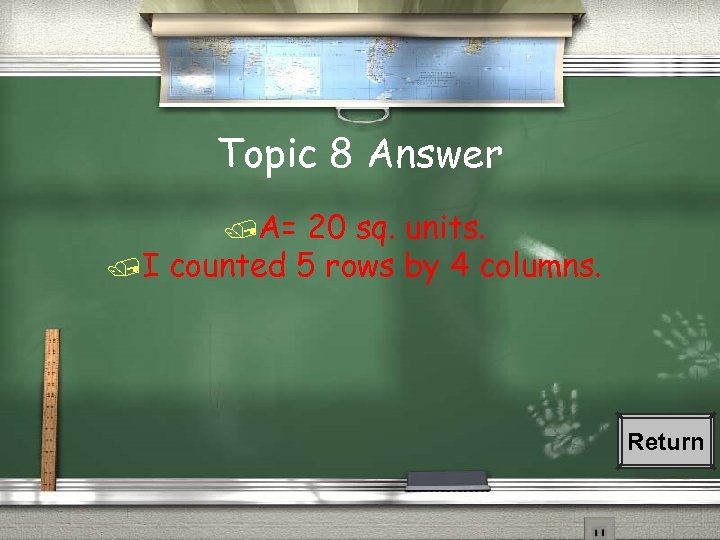Topic 8 Answer /A= 20 sq. units. /I counted 5 rows by 4 columns. Return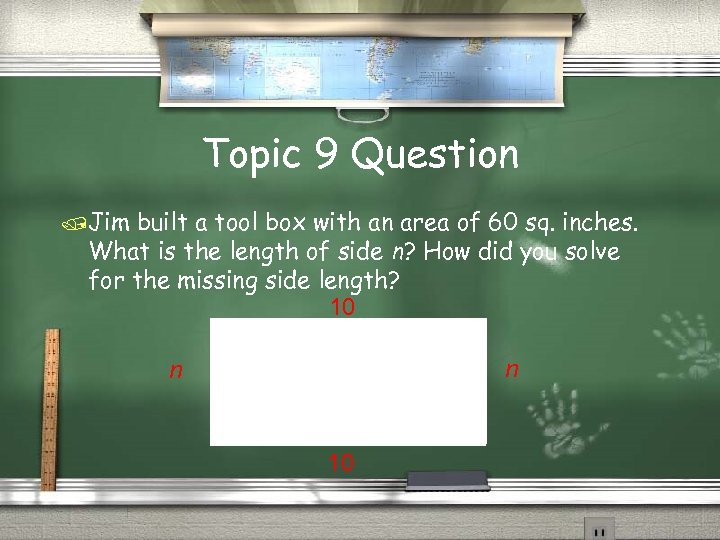Topic 9 Question /Jim built a tool box with an area of 60 sq. inches. What is the length of side n? How did you solve for the missing side length? 10 n n 10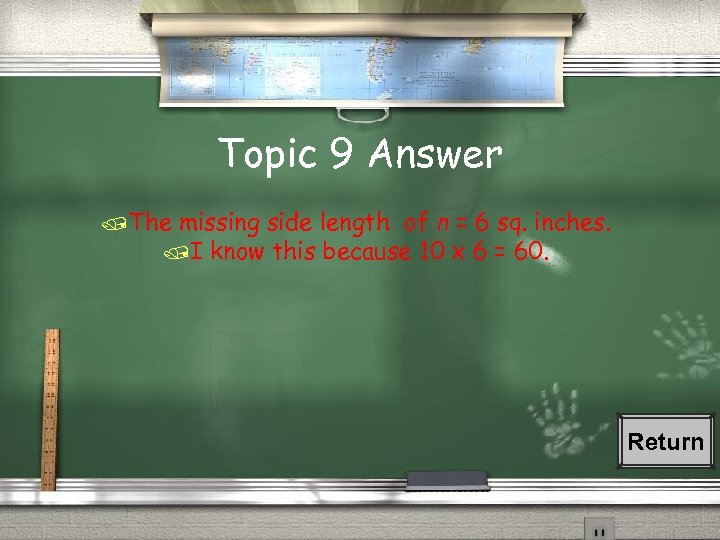Topic 9 Answer /The missing side length of n = 6 sq. inches. /I know this because 10 x 6 = 60. Return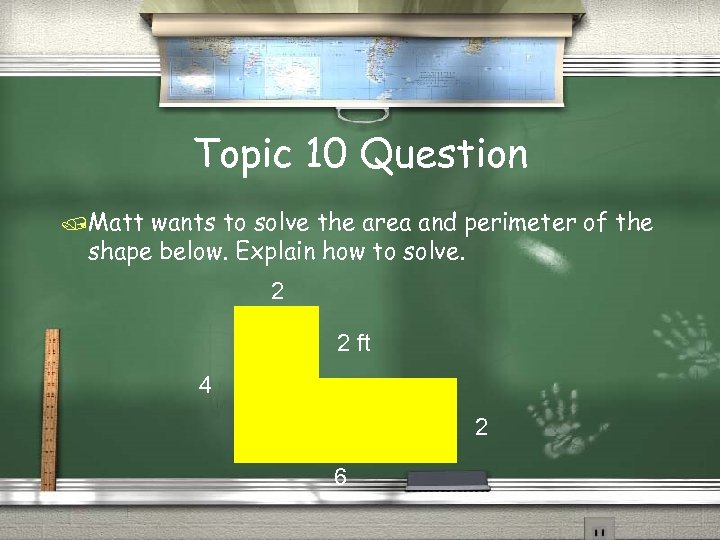Topic 10 Question /Matt wants to solve the area and perimeter of the shape below. Explain how to solve. 2 2 ft 4 2 6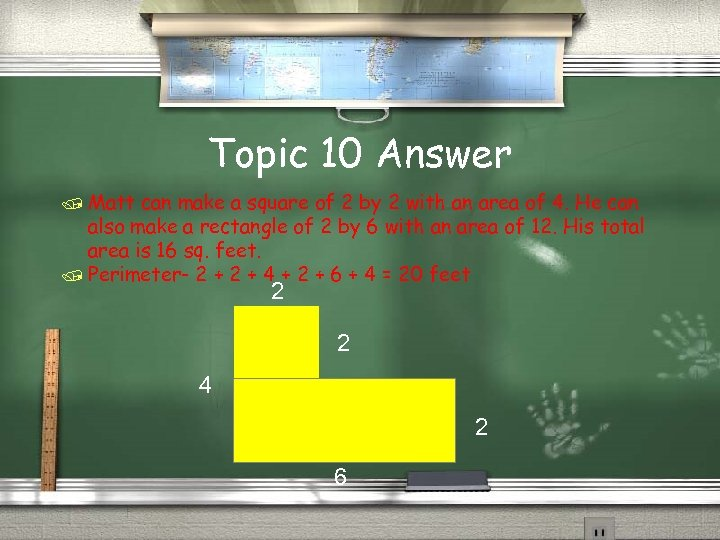Topic 10 Answer / Matt can make a square of 2 by 2 with an area of 4. He can also make a rectangle of 2 by 6 with an area of 12. His total area is 16 sq. feet. / Perimeter- 2 + 4 + 2 + 6 + 4 = 20 feet 2 2 4 2 6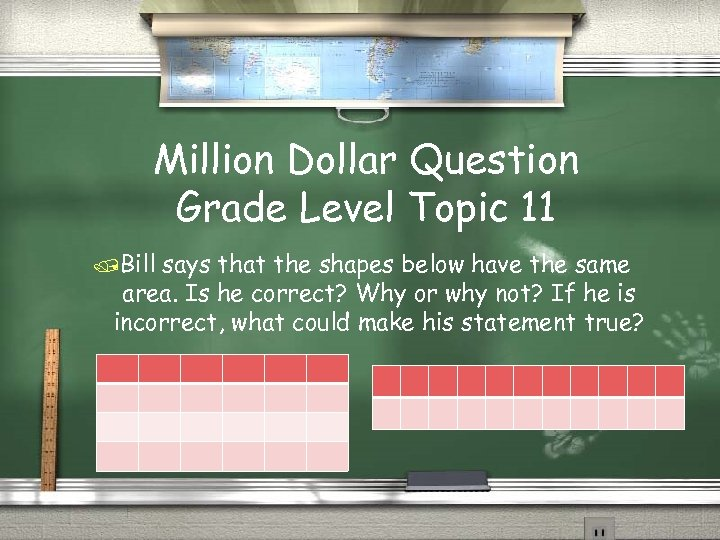Million Dollar Question Grade Level Topic 11 /Bill says that the shapes below have the same area. Is he correct? Why or why not? If he is incorrect, what could make his statement true?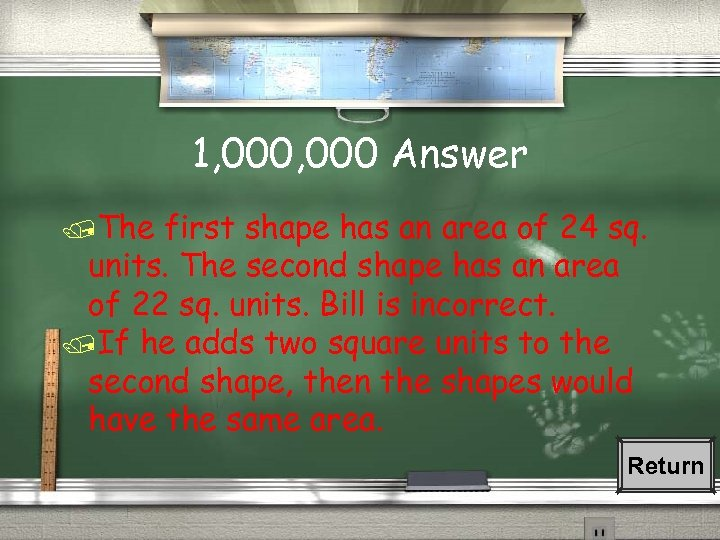1, 000 Answer /The first shape has an area of 24 sq. units. The second shape has an area of 22 sq. units. Bill is incorrect. /If he adds two square units to the second shape, then the shapes would have the same area. Return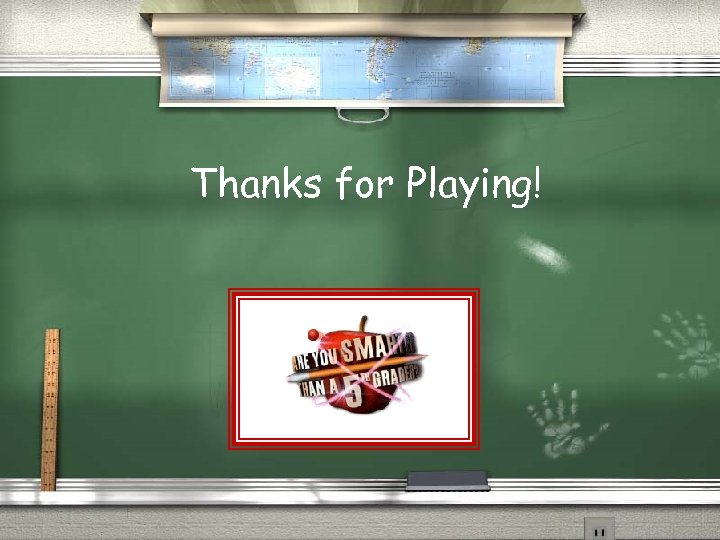Thanks for Playing!